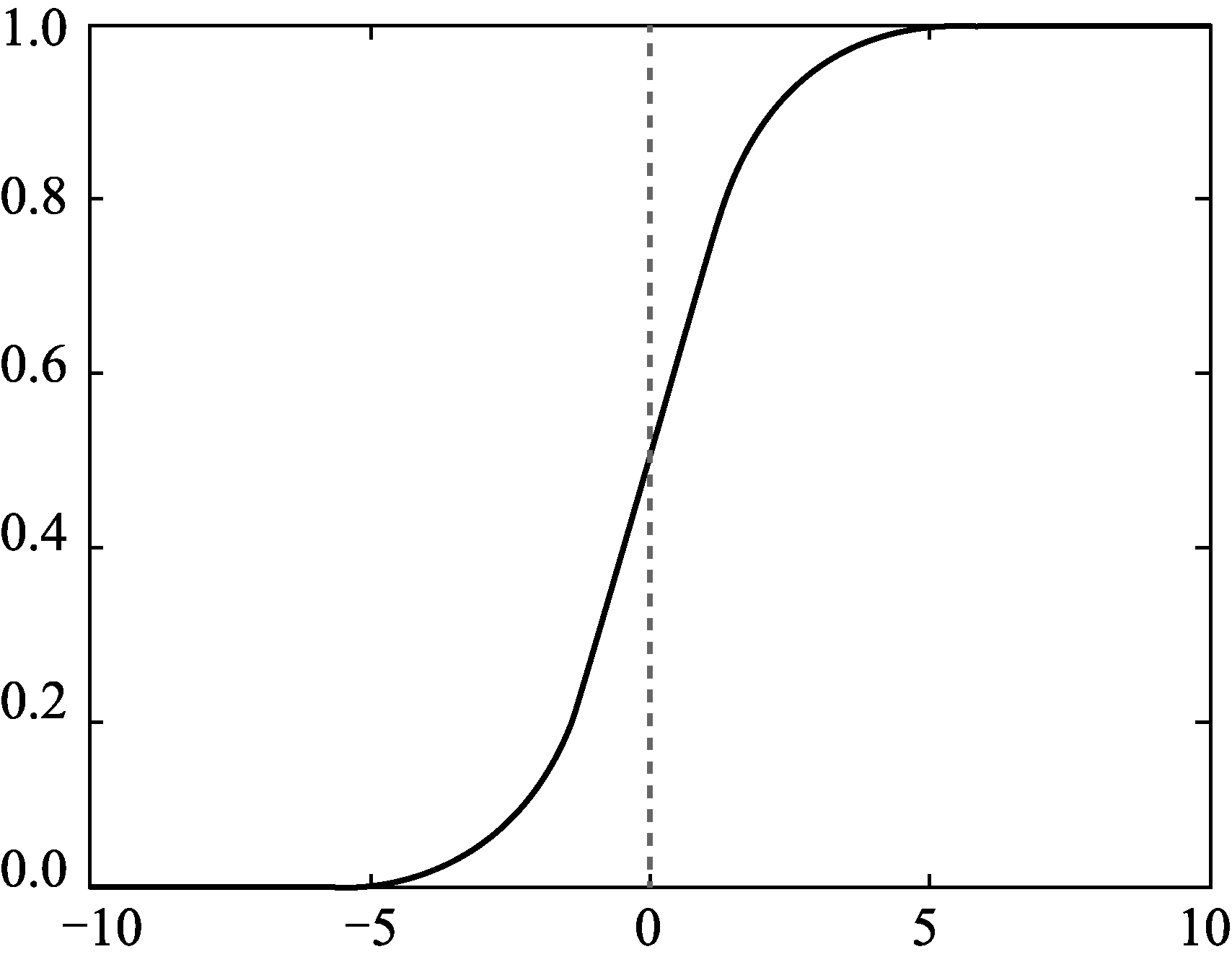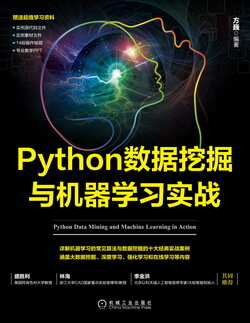# Python 数据挖掘与机器学习实战 (70)：回归分析介 3.6.1Python 数据挖掘与机器学习实战 (70)：回归分析介 3.6.1

(构造预测函数)

$g(z)=\frac{1}{1+{\rm e}^{-z}}\quad\quad\quad\quad\quad\quad\quad(3-11)$$z=\theta^{{\rm T}}x=\theta_0x_0+\theta_1x_1+\dots\dots+\theta_nx_n=\sum\limits^n_{i=0}\theta_ix_i\quad\quad\quad\quad\quad\quad\quad(3-12)$

$\theta=[\theta_0,\theta_1,\theta_2,\dots,\theta_n]^{{\rm T}}\quad\quad\quad\quad\quad\quad\quad(3-13)$

$h_{\theta}(x)=g(\theta^{{\rm T}}x)=\frac{1}{1+{\rm e}^{-\theta^{{\rm T}}x}}\quad\quad\quad\quad\quad\quad\quad(3-14)$

sigmoid 的函数输出是介于 (0,1) 之间的，中间值是 0.5，公式 $h_{\theta}(x)$ 的含义就很好理解了，因为 $h_{\theta}(x)$ 输出是介于 (0,1）之间，也就表明了数据属于某一类别的概率。例如，$h_{\theta}(x)<0.5$ 则说明当前数据属于 A 类；$h_{\theta}(x)>0.5$ 则说明当前数据属于 B 类。所以可以将 sigmoid 函数看成样本数据的概率密度函数。

$p(y=1|x;\theta)=h_{\theta}(x)\quad\quad\quad\quad\quad\quad\quad(3-15)$

$p(y=0|x;\theta)=1-h_{\theta}(x)\quad\quad\quad\quad\quad\quad\quad(3-16)$